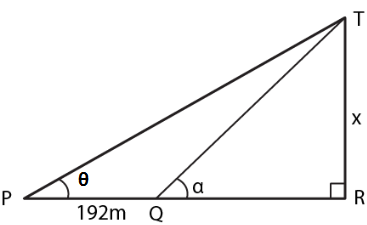Guru

# At a point on level ground, the angle of elevation of a vertical lower is found to be such that its tangent is 5/12. On walking 192 m towards the tower, the tangent of the angle is found to be ¾. Find the height of the tower.

• 0

sir this is the question from the book -ML aggarwal( avichal publication) class 10th , chapter20 , heights and distances
we have been given that At a point on level ground,
the angle of elevation of a vertical lower is found to be such that its tangent is 5/12.

On walking 192 m towards the tower, the tangent of the angle is found to be ¾.
wehave to Find the height of the tower.

question no 21 , heights and distances , ICSE board, ML Aggarwal

Share

1. Consider TR as the tower and P as the point on the ground such that tan θ = 5/12

tan α = ¾

PQ = 192 m

Take TR = x and QR = yIn right triangle TQR

tan α = TR/QR = x\y

So we get

3/4 = x/y

y = 4/3 x …..(1)

In right triangle TPR

tan θ = TR/PR

Substituting the values

5/12 = x/ (y + 192)

x = (y + 192) 5/12 …… (2)

Using both the equations

x = (4/3 x + 192) 5/12

So we get

x = 5/9x + 80

x – 5/9 x = 80

4/9 x = 80

By further calculation

x = (80 × 9)/ 4 = 180

Hence, the height of the tower is 180 m.

• 0